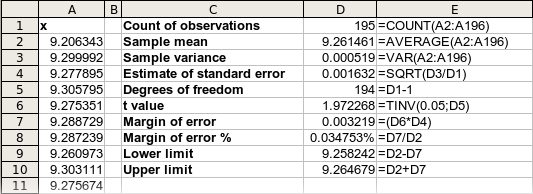Calculating a confidence interval - OpenOffice.org Ninja

# Calculating a confidence interval

#### Posted by Andrew Z at Sunday, December 23, 2007 | PermalinkWhen working with statistics, OpenOffice.org Calc is handy to calculate a confidence interval. The purpose is to estimate the mean (average) of a population from a sample of the population.

As a typical example CNN reported, "63 percent of respondents say [New Orleans] should rebuild" after Hurricane Katrina (September 2005). A small note at the bottom of the article indicates the confidence level: "For poll results based on the total sample, one can say with 95 percent confidence that the margin of error is plus or minus 4 percentage points." CNN could not poll everyone, so they polled a sample. Then, based on the data, they were able to estimate how well the sample (those polled in New Orleans) represents the population (everyone in New Orleans).

## Checklist

Your data must be a random sample.

## Getting data

For our tutorial, let's use the NIST data set Heat Flow Meter 1. You may substitute your own data with the proper modifications to the formulas (more on that later).

1. Open a blank OpenOffice.org Calc document.
2. In cell A1, type x to label the column.
3. Visit NIST's Heat Flow Meter data set page.
4. Copy the 195 observations to the clipboard.
6. In cell A2, paste (using the toolbar or the keyboard shortcut CTRL+V).

### Calculating the confidence interval

These formulas calculate the confidence interval and some dependent univariate statistics.
1. In cell C1, type Count of observations.
2. In cell D1, type =COUNT(A2:A196) .
3. In cell C2, type Sample mean .
4. In cell D2, type =AVERAGE(A2:A196) .
5. In cell C3, type Sample variance .
6. In cell D3, type =VAR(A2:A196) .
7. In cell C4, type Estimate of standard error .
8. In cell D4, type =SQRT(D3/D1) .
9. In cell C5, type Degrees of freedom .
10. In cell D5, type =D1-1 .
11. In cell C6, type t value .
12. In cell D6, type =TINV(0.05;D5) . This is the value of the inverse t-distribution. This calculation saves you a trip to your book's t-tables. Note that 0.05 (called alpha) = 100% - 95%.
13. In cell C7, type Margin of error .
14. In cell D7, type =(D6*D4) .
15. In cell C8, type Margin of error % .
16. In cell D8, type =D7/D2 .
17. In cell D8, press the Number Format: Percent button on the toolbar.
18. In cell C9, type Lower limit .
19. In cell D9, type =D2-D7 .
20. In cell C10, type Upper limit .
21. In cell D10, type =D2+D7 .

If you use a data set with more or less than 195 observations, you would need to adjust A196 in the above formulas to refer to the last cell.

Here the final results. Column E shows the formulas in column D.## Exercises

1. Format the appropriate cells to display 6 decimal places.
2. Adjust the formulas to compute the 99% confidence intervals.
3. Create a histogram chart for the data set.
4. Add the observation 9.278823 to the data set. Adjust the formula so it is included.
5. Why can you not interpret these results to mean, "We are 95% sure that 63% of New Orleans residents think they should rebuild after the hurricane"?
6. Click on each formula in the D column. Then click the Insert > Function menu to bring up the Function Wizard. Then click on each input parameter, and review the help it provides.
7. In OpenOffice.org, open the help by choosing the Help > OpenOffice.org Help menu. In the help index, lookup each of the functions used in column D.
8. Add new formulas to calculate the median, maximum, minimum, range, and standard deviation.
9. Add new formulas to calculate these descriptive statistics: kurtosis and skewness.
10. Why can you not use the formula =CONFIDENCE(0.05;STDEV(A2:A196);195) to calculate the confidence intervals in this example?

### Microsoft Excel 2003

This procedure is the same in Excel 2003 except that Excel uses commas do delimit parameters in formulas where OpenOffice.org uses semicolons. No retraining is required.

Online Statistics: Confidence Interval for the Mean

laurel said...

This is awesome. I was ready to throw my intro to engineering book across the room but your blog made me laugh so now I won't.

Anonymous said...

Thanks dude, Stat class just got easier.

Mikael said...

Where can I download this document, so I don't have to enter all the formulas manually?

Andrew Z said...

Mikael: That's a good question. I never kept the document and didn't publish it either, so you'll have to make it once and save your own copy.